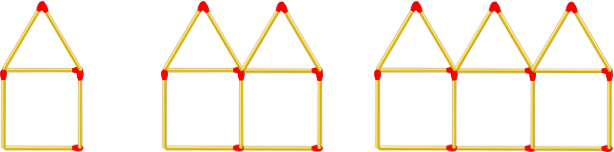# Java Program for Matchstick Houses | Java Programs

Java program for matchstick houses, This challenge will help you interpret mathematical relationships both algebraically and geometrically.

Create a function that takes a number (step) as an argument and returns the amount of matchsticks in that step. See step 1, 2 and 3 in the image above.

Examples:

• matchHouses(1) ➞ 6
• matchHouses(4) ➞ 21
• matchHouses(87) ➞ 436

To find the number of matchsticks required to make the houses, we will first require the number of houses to be made (n).

Since, the number of houses can keep varying based on our testcase and requirement, it is advisable to read this input at runtime. So, to read it at runtime, we make use of a very well-known class named Scanner class.This class, reads input of any primitive datatype like int, float, double, string,etc., at runtime. To make use of this class, we must initially create an object to instantiate this class and then make use of this object to invoke the method based on our datatype.

Here as our input is number of houses, it is definitely an integer. So, we will invoke the nextInt() method using the object as follows:

With the required input in hand, we will make a method call to a static method (MH) by passing this input as argument. This static method (MH) consists of the set of statements to arrive out our solution. In this method (MH), we will first initialize the sum variable (s) to 6.

This is because this is the minimum number of matchsticks required to construct a single house. If the number of houses to be constructed is less than one then, we return zero as our output to the main method.

int s=6;

if(n<1)

return 0;

Else, the we make use of an equation to find the number of sticks required. Since, s=6 so, by making use of the below formula, we add it further to the same sum variable (s). The equation is as given below:

else {

s+=(n-1)*5;

return s;

}

This final value s is, our resultant output which denotes the number of sticks required to construct n houses.

Output:

If number of houses n=87 then,

s=6+(87-1)*5=6+430=436

If number of houses n=54 then,

s=6+(54-1)*5=6+265=271

If number of houses n=4 then,

s=6+(4-1)*5=6+15=21.

x

## Java Interview Questions : For Beginners With Sample Examples

What is instance actually mean in line “An Object is instance of class.”.? Instance ...